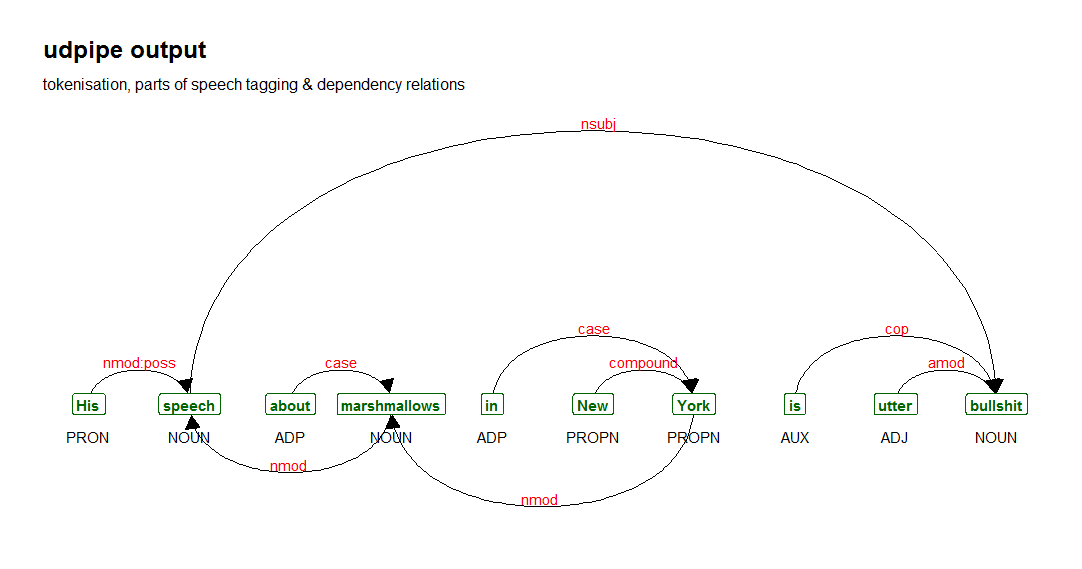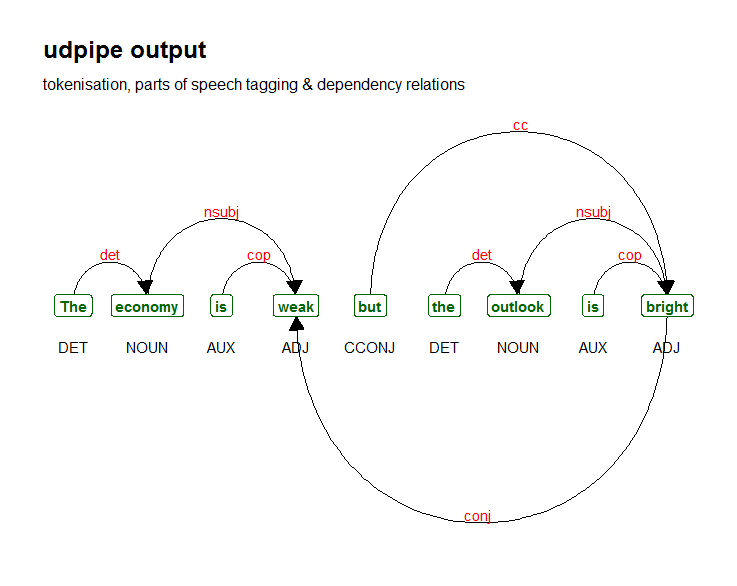# dependency parsing with udpipe

We have been blogging about udpipe several times now in the following posts:

### Dependency parsing

A point which we haven't touched upon yet too much was dependency parsing. Dependency parsing is an NLP technique which provides to each word in a sentence the link to another word in the sentence, which is called it's syntactical head. This link between each 2 words furthermore has a certain type of relationship giving you further details about it.

The R package udpipe provides such a dependency parser. With the output of dependency parsing, you can answer questions like

1. What is the nominal subject of a text
2. What is the object of a verb
3. Which word modifies a noun
4. What is the linked to negative words
5. Which words are compound statements
6. What are noun phrases, verb phrases in the text

### Examples

In the following sentence:

His speech about marshmallows in New York is utter bullshit

you can see this dependency parsing in action in the graph below. You can see compound statement like 'New York', that the word speech is linked to the word bullshit with relationship nominal subject, that the 2 nominals marshmallow and speech are linked as nominal noun modifiers, that the word utter is an adjective which modifies the noun bullshit.Obtaining such relationships in R is pretty simple nowadays. Running this code, will provide you the dependency relationships among the words of the sentence in the columns token_id, head_token_id and dep_rel. The possible values in the field dep_rel are defined at https://universaldependencies.org/u/dep/index.html.

``library(udpipe)``x <- udpipe("His speech about marshmallows in New York is utter bullshit", "english")``R is excellent in visualisation. For visualising the relationships between the words which were found, you can just use the ggraph R package. Below we create a basic function which selects the right columns from the annotation and puts it into a graph.

`library(igraph)library(ggraph)library(ggplot2)plot_annotation <- function(x, size = 3){  stopifnot(is.data.frame(x) & all(c("sentence_id", "token_id", "head_token_id", "dep_rel",                                     "token_id", "token", "lemma", "upos", "xpos", "feats") %in% colnames(x)))  x <- x[!is.na(x\$head_token_id), ]  x <- x[x\$sentence_id %in% min(x\$sentence_id), ]  edges <- x[x\$head_token_id != 0, c("token_id", "head_token_id", "dep_rel")]  edges\$label <- edges\$dep_rel  g <- graph_from_data_frame(edges,                             vertices = x[, c("token_id", "token", "lemma", "upos", "xpos", "feats")],                             directed = TRUE)  ggraph(g, layout = "linear") +    geom_edge_arc(ggplot2::aes(label = dep_rel, vjust = -0.20),                  arrow = grid::arrow(length = unit(4, 'mm'), ends = "last", type = "closed"),                  end_cap = ggraph::label_rect("wordswordswords"),                  label_colour = "red", check_overlap = TRUE, label_size = size) +    geom_node_label(ggplot2::aes(label = token), col = "darkgreen", size = size, fontface = "bold") +    geom_node_text(ggplot2::aes(label = upos), nudge_y = -0.35, size = size) +    theme_graph(base_family = "Arial Narrow") +    labs(title = "udpipe output", subtitle = "tokenisation, parts of speech tagging & dependency relations")}`

We can now call the function as follows to get the plot shown above:

``plot_annotation(x, size = 4)``

Let us see what is gives with the following sentence.

The economy is weak but the outlook is bright

``x <- udpipe("The economy is weak but the outlook is bright", "english")``plot_annotation(x, size = 4)``You can see that with dependency parsing you can now answer the question 'What is weak?', it is the economy. 'What is bright?', it is the outlook as these nouns relate to the adjectives with nominal subject as type of relationship. That's a lot more rich information than just looking at wordclouds.

Hope this has triggered beginning users of natural language processing that there is a myriad of NLP options beyond mere frequency based word counting. Enjoy!